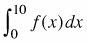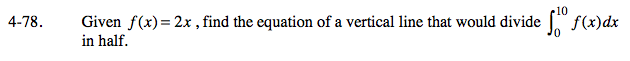### Home > CALC > Chapter 4 > Lesson 4.2.3 > Problem4-78

4-78.

Given f(x) = 2x, find the equation of a vertical line that would dividein half. Homework Help ✎Sketch a graph of

Find the area under the graph from [0, 10] through integration.

Because you want to find the equation that would divide the area of the graph in half, you must solve the following equation:

Use the eTool below to examine the graph.
Click the link at right for the full version of the eTool: Calc 4-78 HW eTool